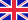•# Advanced Forecasting Techniques with Application to NN5 Time Series

Autor: Robert Andrawis

In this book we analyze the forecasting model that achieved the first rank in the Forecasting Competition for Artificial Neural Networks & Computational Intelligence NN5. The model is based on combination of machine learning and linear models. In addition,... Viac o knihe

Na objednávku, dodanie 2-4 týždne

51.60 €

bežná cena: 60.70 €

## O knihe

In this book we analyze the forecasting model that achieved the first rank in the Forecasting Competition for Artificial Neural Networks & Computational Intelligence NN5. The model is based on combination of machine learning and linear models. In addition, the approach and the experiments done to develop this model are explained in details to allow the reader to learn the methodology of developing such optimal models. The book also introduces a Bayesian forecasting approach for Holt's additive exponential smoothing method. Starting from the state space formulation, a formula for the forecast is derived and reduced to a two-dimensional integration that can be computed numerically in a straightforward way. In contrast with much of the work for exponential smoothing, this method produces the forecast density as well. The combinations of forecast are investigated as well in this book. A comparison between different combination methods is introduced with complete case study on tourism demand forecasting in Egypt.

• Vydavateľstvo: LAP LAMBERT Academic Publishing
• Rok vydania: 2012
• Formát: Paperback
• Rozmer: 220 x 150 mm
• Jazyk: Anglický jazyk
• ISBN: 9783659129629

Generuje redakčný systém BUXUS CMS spoločnosti ui42.